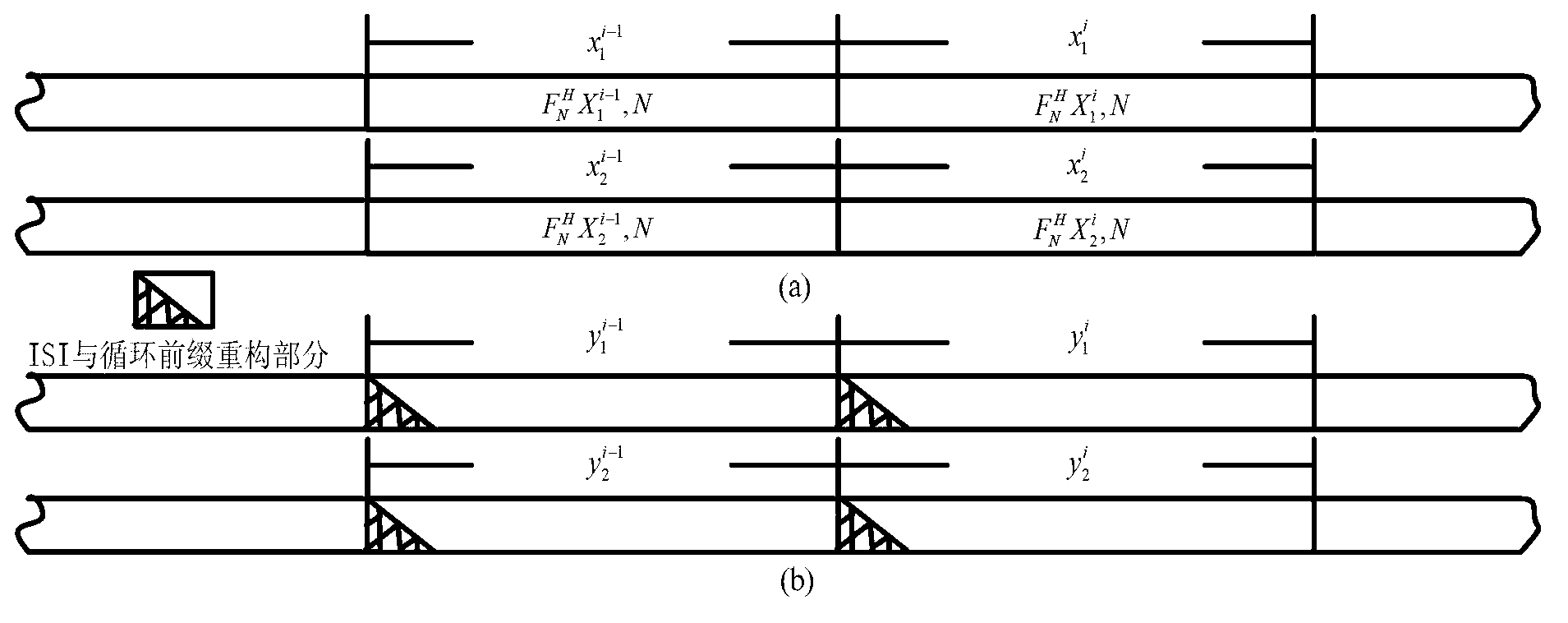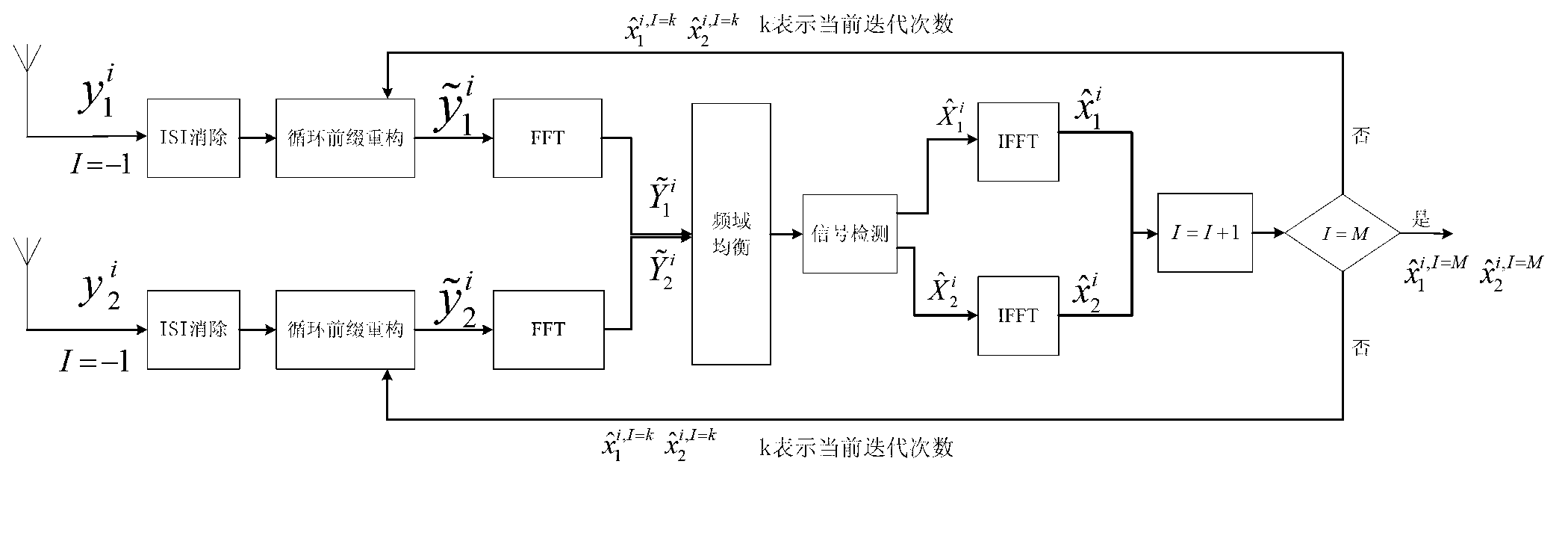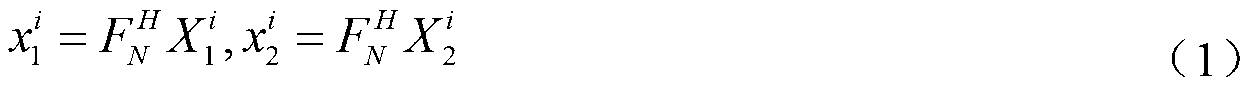# Signal detecting method of VBLAST-OFDM system under condition of shortage of cyclic prefix

## A VBLAST-OFDM, cyclic prefix technology used in wireless and mobile communications

Inactive Publication Date: 2013-08-21
UNIV OF ELECTRONIC SCI & TECH OF CHINA
1 Cites 0 Cited by

## AI-Extracted Technical Summary

### Problems solved by technology

However, these methods are only used in single-transmit and single-receive systems. For the two-transm...
View more

## Abstract

The invention discloses a signal detecting method of a VBLAST-OFDM system under the condition of shortage of a cyclic prefix, and belongs to the technical field of wireless communication. Inter-symbol interference and reconfiguration of cyclic convolution are removed, so that an OPDM transmitting system is divided into N equal gauss channels which are concurrent and different in gain, and then a zero-forcing standard or a minimum mean square error standard is used for completing detection to a VBLAST-OFDM symbol. Directing at the VBLAST-OFDM system which conducts twice sending and twice reception, according to the difference of information channel parameters between antennas, inter-symbol interference elimination and the initial approximation of the cyclic convolution are conducted on two receiving antennas respectively, then the zero-forcing standard or the minimum mean square error standard is used for conducting simplification on a space frequency domain channel matrix, inter-carrier interference and inter-antenna interface are removed, data detection to the VBLAST-OFDM system is completed, finally the cyclic prefix of the data of the two receiving antennas is repaired through iteration, and precision of data detection is improved.

Application Domain

Baseband system detailsMulti-frequency code systems +1

Technology Topic

Channel parameterFrequency domain +11

## Image

•••## Examples

• Experimental program(1)

### Example Embodiment

 detailed description
 The VBLAST-OFDM symbol detection method in the absence of a cyclic prefix proposed by the present invention will be described below in conjunction with specific parameters:
 The parameters of the VBLAST-OFDM system in this example are set as follows: Set the antenna parameters as two transmissions and two receptions, the signal modulation method adopts QPSK modulation, the total number of subcarriers N=1024, and the subcarrier sequence number is [0,1,2,... ,1023]. The channel parameters selected in this example are as follows: The spatial channels use independent Rayleigh fading channels, each channel uses COST207TU channels, and the multipath delays are [0, 0.2, 0.5, 1.6, 2.3, 5] us, and more The path fading power is [3, 0, 5, 6, 8, 10] dB, the normalized Doppler spread value is 0.001, and the system sampling period T = 1.0e-7s. Then the normalized channel maximum multipath delay (number of multipath channels) L=5.0e-6/T=50.
 The signal detection method of the VBLAST-OFDM system in the absence of a cyclic prefix specifically includes the following steps:
 S1: Since the channel state information can be obtained through channel estimation, the receiving end has completed the detection of the i-1th frame data of the two transmitting antennas, so it can be assumed that these two quantities are known; use the known channel state information and Data of the previous frame, get the data of receiving antenna 1 Inter-symbol interference And receive antenna 2 data Inter-symbol interference
 Suppose the frequency domain OFDM symbol transmitted by the i-th frame of the transmitting antenna 1 is The frequency domain OFDM symbol transmitted by the i-th frame of the transmitting antenna 2 is For frequency domain symbols As an IFFT transform of length N, the time domain symbol of the transmitting antenna of the i-th frame can be expressed as,
 x 1 i = F N H X 1 i , x 2 i = F N H X 2 i - - - ( 1 )
 The received data of the i-th frame of the receiving antenna 1 is expressed as In a slow time-varying channel, the channel CIR is basically unchanged during the data duration of two consecutive OFDM symbols, so:
 y 1 , k i = X l = 0 k h 11 , l x 1 , k - 1 N i + X l = 0 k h 12 , l x 2 , k - 1 N i + X l = k + 1 L h 11 , l x l , k - l N i - 1 + X l = k + 1 L h 12 , l x 2 , k - l N i - 1 + n k i 0 ≤ k L X l = 0 L h 11 , l x 1 , k - l N i + X l = 0 L h 12 , l x 2 , k - l N i + n k i L ≤ k N - - - ( 2 )
 Among them, k represents the k+1 sampling point in the OFDM data unit, and N represents the length of the OFDM data unit; Represents the channel information between transmitting antenna 1 and receiving antenna 1, Represents the channel information between the transmitting antenna 2 and the receiving antenna 1, l represents the normalized multipath channel, and L represents the normalized maximum number of multipath signals;
 Then:
 y 1 , Re , ISI i ( k ) = X l = k + 1 L h 11 , l x 1 , k - l N i - 1 + X l = k + 1 L h 12 , l x 2 , k - l N i - 1 0 ≤ k L - - - ( 3 )
 The same can be seen:
 y 2 , Re _ ISI i ( k ) = X l = k + 1 L h twenty one , l x 1 , k - l N i - 1 + X l = k + 1 L h twenty two , l x 2 , k - l N i - 1 0 ≤ k L - - - ( 4 )
 among them, Indicates the channel information between transmitting antenna 1 and receiving antenna 2, Indicates the channel information between the transmitting antenna 2 and the receiving antenna 2;
 S2: Use two receiving antennas to receive data in the i+1th frame with The first L sample pairs with Perform the initial reconstruction of the cyclic prefix, where, Means The part of the data that needs to be reconstructed; Means The part of the data that needs to be reconstructed;
 y 1 , Add i ( k ) = ξ 1 , k y 1 , k i + 1 , 0 ≤ k L - - - ( 5 )
 among them:
 ξ 1 , k = X l = k + 1 L | h 11 , l | 2 + X l = k + 1 L | h 12 , l | 2 X l = 0 L | h 11 , l | 2 + X l = 0 L | h 12 , l | 2 - - - ( 6 )
 The first L samples of can be expressed as:
 y 1 , k i + 1 = X l = 0 k h 11 , l x 1 , k - l N i + 1 + X l = 0 k h 12 , l x 2 , k - 1 N i + 1 + X l = k + 1 L h 11 , l x 1 , k - l N i + X l = k + 1 L h 12 , l x 2 , k - l N i + n 1 , k i 0 ≤ k L - - - ( 7 )
 Similarly, there are:
 y 2 , Add i + 1 ( k ) = ξ 2 , k y 2 , k i + 2 - - - ( 8 )
 among them:
 ξ 2 , k = X l = k + 1 L | h twenty one , l | 2 + X l = k + 1 L | h twenty two , l | 2 X l = 0 L | h twenty one , l | 2 + X l = 0 L | h twenty two , l | 2 0 ≤ k L - - - ( 9 )
 The first L samples of can be expressed as:
 y 2 , k i + 1 = X l = 0 k h twenty one , l x 1 , k - l N i + 1 + X l = 0 k h twenty two , l x 2 , k - l N i + 1 + X l = k + 1 L h twenty one , l x 1 , k - l N i + X l = k + 1 L h twenty two , l x 2 , k - l N i + n 2 , k i 0 ≤ k L - - - ( 10 )
 S3: Yes Perform interference cancellation and initial cyclic prefix reconstruction to obtain
 y ~ 1 , k i = y 1 , k i - y 1 , Re _ ISI i ( k ) + y 1 , Add i ( k ) , 0 ≤ k L y 1 , k i , L ≤ k N - - - ( 11 )
 S4: Yes Perform interference cancellation and initial cyclic prefix reconstruction to obtain
 y ~ 2 , k i = y 2 , k i - y 2 , Re _ ISI i ( k ) + y 2 , Add i ( k ) , 0 ≤ k L y 2 , k i L ≤ k N - - - ( 12 )
 S5: Obtained from step S3 And step S4 Perform Fourier transform to get frequency domain response with
 S6: Channel equalization and signal detection;
 Due to ISI elimination and cyclic prefix reconstruction, the receiving antenna 1 receives the i-th frame of data And receive antenna 2 to receive the i-th frame data It can be approximately expressed as:
 y ~ 1 , k i = h 11 , eqv x 1 i + h 12 , eqv x 2 i - - - ( 13 )
 y ~ 2 , k i = h twenty one , eqv x 1 i + h twenty two , eqv x 2 i - - - ( 14 )
 Where h 11,eqv ,h 12,eqv ,h 21,eqv And h 22,eqv Both are cyclic shift matrices, and the form can be expressed as:
 h eqv = h 0 0 · · · h L · · · h 1 h 1 h 0 · · · · · · · · · h 2 h 2 h 1 h 0 · · · · · · h 3 · · · · · · · · · · · · · · · · · · · · · · · · · · · · · · · · · · · · 0 · · · h L h L - 1 · · · h 0 - - - ( 15 )
 Then the frequency domain response is expressed as:
 Y ~ 1 i = H 11 H 1 i + H 12 X 2 i + W 1 i - - - ( 16 )
 Y ~ 2 i = H twenty one X 1 i + H twenty two X 1 i + W 2 i - - - ( 17 )
 among them:
 H 11 = F N h 11 , eqv F N H , H 12 = F N h 12 , eqv F N H H twenty one = F N h twenty one , eqv F N H , H twenty two = F N h twenty two , eqv F N H - - - ( 18 )
 Here H 11 ,H 12 ,H 21 And H 22 Are diagonal matrices
 make H = H 11 H 12 H twenty one H twenty two , The MMSE criterion is used to eliminate the interference between antennas and obtain the frequency domain equalizer Q:
 Q = H H H H H + σ 2 I - - - ( 19 )
 After the frequency domain equalization is completed, the transmitted signal is determined based on the minimum mean square error criterion with Do N-point IFFT to get the transmitted time domain signal with
 S7: Yes The cyclic prefix reconstruction part is revised, as follows:
 y ~ 1 , k i , I = 1 = y 1 , k i - y 1 , Re _ ISI i ( k ) + y 1 , Add i , I = 1 ( k ) , 0 ≤ k L y 1 , k i , L ≤ k N - - - ( 20 )
 among them:
 y 1 , Add i , I = 1 ( k ) = X l = k + 1 L h 11 , l x ^ 1 , k - l N i + X l = k + 1 L h 12 , l x ^ 2 , k - l N i 0 ≤ k L - - - ( twenty one )
 S8: Yes Perform interference cancellation and cyclic prefix correction, expressed as:
 y ~ 2 , k i , I = 1 = y 2 , k i - y 2 , Re _ ISI i ( k ) + y 2 , Add i , I = 1 ( k ) , 0 ≤ k L y 2 , k i , L ≤ k N - - - ( twenty two )
 among them:
 y 2 , Add i , I = 1 ( k ) = X l = k + 1 L h twenty one , l x ^ 1 , k - l N i + X l = k + 1 L h twenty two , l x ^ 2 , k - l N i 0 ≤ k L - - - ( twenty three )
 S9: Update;
 Use the updated received data response after iteration with Return to step S5 and repeat the above process to obtain the iterative detection data with Continue to iterate until it reaches the preset number of iterations M, and record the final test data as with x ^ 2 i , I = M .
 The present invention proposes to realize the detection of VBLAST-OFDM symbols through inter-symbol interference elimination and reconstruction cyclic convolution. In step S2, the interference cancellation items and the cyclic prefix reconstruction items corresponding to the number of the two receiving antennas are respectively calculated, and in step S4, the cyclic prefix reconstruction of the two receiving antenna data is corrected respectively.

## PUM## Description & Claims & Application Information

We can also present the details of the Description, Claims and Application information to help users get a comprehensive understanding of the technical details of the patent, such as background art, summary of invention, brief description of drawings, description of embodiments, and other original content. On the other hand, users can also determine the specific scope of protection of the technology through the list of claims; as well as understand the changes in the life cycle of the technology with the presentation of the patent timeline. Login to view more.
Who we serve
• R&D Engineer
• R&D Manager
• IP Professional
Why Eureka